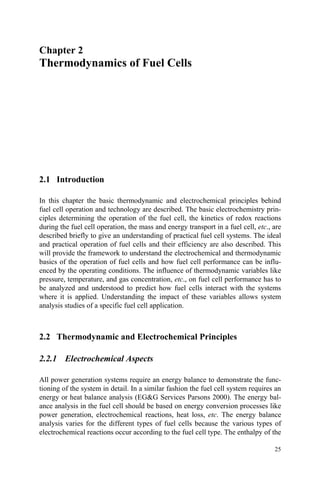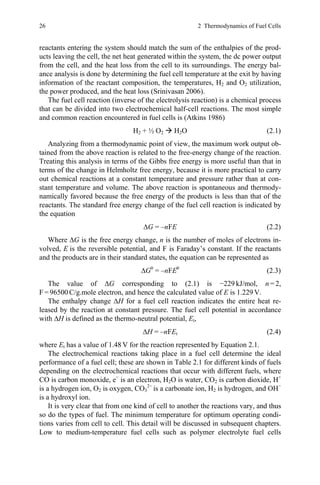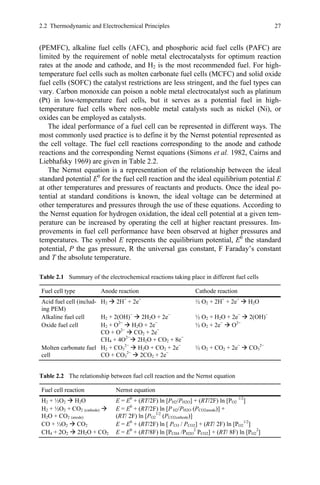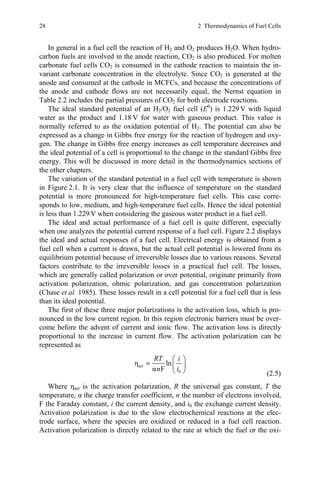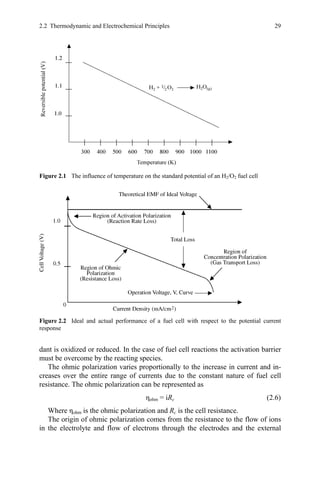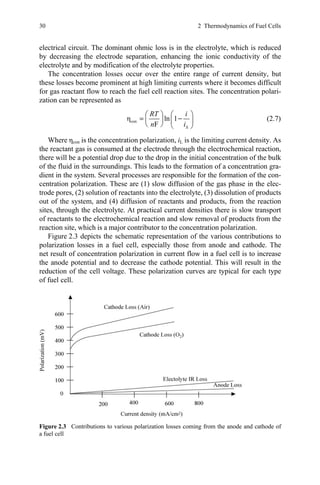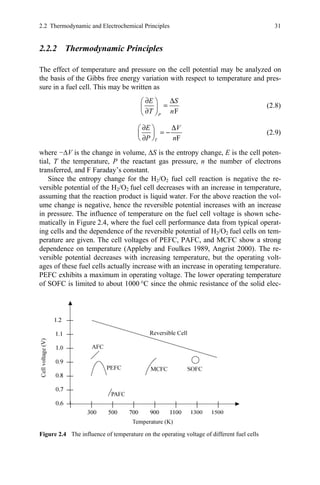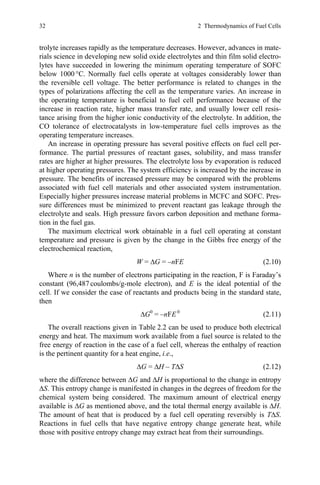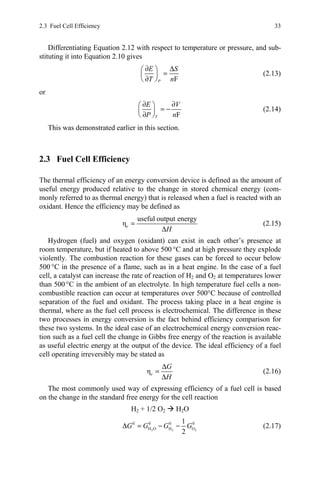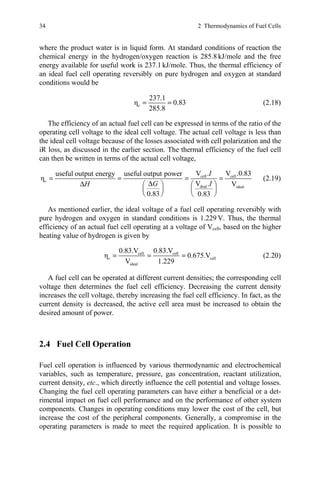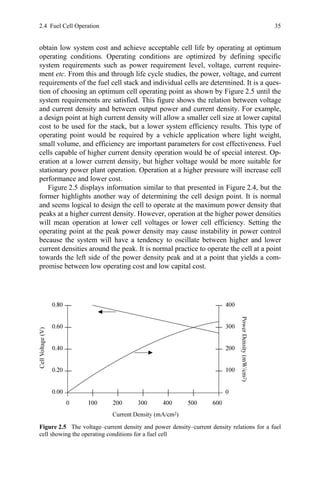Próximo SlideShare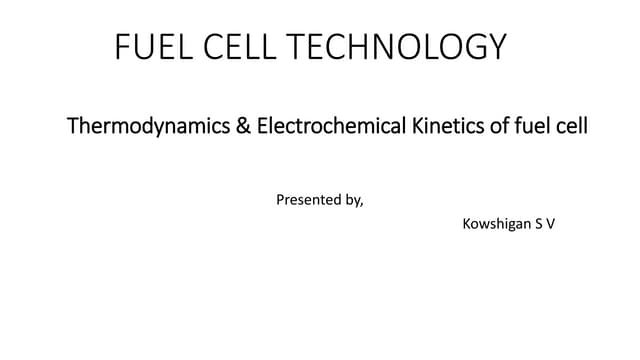Thermodynamics &amp; electrochemical kinetics of fuel cell
Cargando en ... 3
1 de 13

### Energy balance.pdf

1. 25 Chapter 2 Thermodynamics of Fuel Cells 2.1 Introduction In this chapter the basic thermodynamic and electrochemical principles behind fuel cell operation and technology are described. The basic electrochemistry prin- ciples determining the operation of the fuel cell, the kinetics of redox reactions during the fuel cell operation, the mass and energy transport in a fuel cell, etc., are described briefly to give an understanding of practical fuel cell systems. The ideal and practical operation of fuel cells and their efficiency are also described. This will provide the framework to understand the electrochemical and thermodynamic basics of the operation of fuel cells and how fuel cell performance can be influ- enced by the operating conditions. The influence of thermodynamic variables like pressure, temperature, and gas concentration, etc., on fuel cell performance has to be analyzed and understood to predict how fuel cells interact with the systems where it is applied. Understanding the impact of these variables allows system analysis studies of a specific fuel cell application. 2.2 Thermodynamic and Electrochemical Principles 2.2.1 Electrochemical Aspects All power generation systems require an energy balance to demonstrate the func- tioning of the system in detail. In a similar fashion the fuel cell system requires an energy or heat balance analysis (EG&G Services Parsons 2000). The energy bal- ance analysis in the fuel cell should be based on energy conversion processes like power generation, electrochemical reactions, heat loss, etc. The energy balance analysis varies for the different types of fuel cells because the various types of electrochemical reactions occur according to the fuel cell type. The enthalpy of the
2. 26 2 Thermodynamics of Fuel Cells reactants entering the system should match the sum of the enthalpies of the prod- ucts leaving the cell, the net heat generated within the system, the dc power output from the cell, and the heat loss from the cell to its surroundings. The energy bal- ance analysis is done by determining the fuel cell temperature at the exit by having information of the reactant composition, the temperatures, H2 and O2 utilization, the power produced, and the heat loss (Srinivasan 2006). The fuel cell reaction (inverse of the electrolysis reaction) is a chemical process that can be divided into two electrochemical half-cell reactions. The most simple and common reaction encountered in fuel cells is (Atkins 1986) H2 + ½ O2 Æ H2O (2.1) Analyzing from a thermodynamic point of view, the maximum work output ob- tained from the above reaction is related to the free-energy change of the reaction. Treating this analysis in terms of the Gibbs free energy is more useful than that in terms of the change in Helmholtz free energy, because it is more practical to carry out chemical reactions at a constant temperature and pressure rather than at con- stant temperature and volume. The above reaction is spontaneous and thermody- namically favored because the free energy of the products is less than that of the reactants. The standard free energy change of the fuel cell reaction is indicated by the equation ∆G = –nFE (2.2) Where ∆G is the free energy change, n is the number of moles of electrons in- volved, E is the reversible potential, and F is Faraday’s constant. If the reactants and the products are in their standard states, the equation can be represented as ∆G0 = –nFE0 (2.3) The value of ∆G corresponding to (2.1) is −229 kJ/mol, n = 2, F = 96500 C/g.mole electron, and hence the calculated value of E is 1.229 V. The enthalpy change ∆H for a fuel cell reaction indicates the entire heat re- leased by the reaction at constant pressure. The fuel cell potential in accordance with ∆H is defined as the thermo-neutral potential, Et, ∆H = –nFEt (2.4) where Et has a value of 1.48 V for the reaction represented by Equation 2.1. The electrochemical reactions taking place in a fuel cell determine the ideal performance of a fuel cell; these are shown in Table 2.1 for different kinds of fuels depending on the electrochemical reactions that occur with different fuels, where CO is carbon monoxide, e− is an electron, H2O is water, CO2 is carbon dioxide, H+ is a hydrogen ion, O2 is oxygen, CO3 2− is a carbonate ion, H2 is hydrogen, and OH− is a hydroxyl ion. It is very clear that from one kind of cell to another the reactions vary, and thus so do the types of fuel. The minimum temperature for optimum operating condi- tions varies from cell to cell. This detail will be discussed in subsequent chapters. Low to medium-temperature fuel cells such as polymer electrolyte fuel cells
3. 2.2 Thermodynamic and Electrochemical Principles 27 (PEMFC), alkaline fuel cells (AFC), and phosphoric acid fuel cells (PAFC) are limited by the requirement of noble metal electrocatalysts for optimum reaction rates at the anode and cathode, and H2 is the most recommended fuel. For high- temperature fuel cells such as molten carbonate fuel cells (MCFC) and solid oxide fuel cells (SOFC) the catalyst restrictions are less stringent, and the fuel types can vary. Carbon monoxide can poison a noble metal electrocatalyst such as platinum (Pt) in low-temperature fuel cells, but it serves as a potential fuel in high- temperature fuel cells where non-noble metal catalysts such as nickel (Ni), or oxides can be employed as catalysts. The ideal performance of a fuel cell can be represented in different ways. The most commonly used practice is to define it by the Nernst potential represented as the cell voltage. The fuel cell reactions corresponding to the anode and cathode reactions and the corresponding Nernst equations (Simons et al. 1982, Cairns and Liebhafsky 1969) are given in Table 2.2. The Nernst equation is a representation of the relationship between the ideal standard potential E0 for the fuel cell reaction and the ideal equilibrium potential E at other temperatures and pressures of reactants and products. Once the ideal po- tential at standard conditions is known, the ideal voltage can be determined at other temperatures and pressures through the use of these equations. According to the Nernst equation for hydrogen oxidation, the ideal cell potential at a given tem- perature can be increased by operating the cell at higher reactant pressures. Im- provements in fuel cell performance have been observed at higher pressures and temperatures. The symbol E represents the equilibrium potential, E0 the standard potential, P the gas pressure, R the universal gas constant, F Faraday’s constant and T the absolute temperature. Table 2.1 Summary of the electrochemical reactions taking place in different fuel cells Fuel cell type Anode reaction Cathode reaction Acid fuel cell (includ- ing PEM) H2 Æ 2H+ + 2e+ ½ O2 + 2H+ + 2e+ Æ H2O Alkaline fuel cell H2 + 2(OH)− Æ 2H2O + 2e− ½ O2 + H2O + 2e− Æ 2(OH)− Oxide fuel cell H2 + O2− Æ H2O + 2e− CO + O2− Æ CO2 + 2e− CH4 + 4O2− Æ 2H2O + CO2 + 8e− ½ O2 + 2e− Æ O2− Molten carbonate fuel cell H2 + CO3 2− Æ H2O + CO2 + 2e− CO + CO3 2− Æ 2CO2 + 2e− ½ O2 + CO2 + 2e− Æ CO3 2− Table 2.2 The relationship between fuel cell reaction and the Nernst equation Fuel cell reaction Nernst equation H2 + ½O2 Æ H2O E = E0 + (RT/2F) ln [PH2/PH2O] + (RT/2F) ln [PO2 1/2 ] H2 + ½O2 + CO2 (cathode) Æ H2O + CO2 (anode) E = E0 + (RT/2F) ln [P H2/PH2O (PCO2anode)] + (RT/ 2F) ln [PO2 1/2 (PCO2cathode)] CO + ½O2 Æ CO2 E = E0 + (RT/2F) ln [ PCO / PCO2] + (RT/ 2F) ln [PO2 1/2 ] CH4 + 2O2 Æ 2H2O + CO2 E = E0 + (RT/8F) ln [PCH4 /PH2O 2 PCO2] + (RT/ 8F) ln [PO2 2 ]
4. 28 2 Thermodynamics of Fuel Cells In general in a fuel cell the reaction of H2 and O2 produces H2O. When hydro- carbon fuels are involved in the anode reaction, CO2 is also produced. For molten carbonate fuel cells CO2 is consumed in the cathode reaction to maintain the in- variant carbonate concentration in the electrolyte. Since CO2 is generated at the anode and consumed at the cathode in MCFCs, and because the concentrations of the anode and cathode flows are not necessarily equal, the Nernst equation in Table 2.2 includes the partial pressures of CO2 for both electrode reactions. The ideal standard potential of an H2/O2 fuel cell (E0 ) is 1.229 V with liquid water as the product and 1.18 V for water with gaseous product. This value is normally referred to as the oxidation potential of H2. The potential can also be expressed as a change in Gibbs free energy for the reaction of hydrogen and oxy- gen. The change in Gibbs free energy increases as cell temperature decreases and the ideal potential of a cell is proportional to the change in the standard Gibbs free energy. This will be discussed in more detail in the thermodynamics sections of the other chapters. The variation of the standard potential in a fuel cell with temperature is shown in Figure 2.1. It is very clear that the influence of temperature on the standard potential is more pronounced for high-temperature fuel cells. This case corre- sponds to low, medium, and high-temperature fuel cells. Hence the ideal potential is less than 1.229 V when considering the gaseous water product in a fuel cell. The ideal and actual performance of a fuel cell is quite different, especially when one analyzes the potential current response of a fuel cell. Figure 2.2 displays the ideal and actual responses of a fuel cell. Electrical energy is obtained from a fuel cell when a current is drawn, but the actual cell potential is lowered from its equilibrium potential because of irreversible losses due to various reasons. Several factors contribute to the irreversible losses in a practical fuel cell. The losses, which are generally called polarization or over potential, originate primarily from activation polarization, ohmic polarization, and gas concentration polarization (Chase et al. 1985). These losses result in a cell potential for a fuel cell that is less than its ideal potential. The first of these three major polarizations is the activation loss, which is pro- nounced in the low current region. In this region electronic barriers must be over- come before the advent of current and ionic flow. The activation loss is directly proportional to the increase in current flow. The activation polarization can be represented as act 0 η ln α F RT i n i ⎛ ⎞ = ⎜ ⎟ ⎝ ⎠ (2.5) Where ηact is the activation polarization, R the universal gas constant, T the temperature, α the charge transfer coefficient, n the number of electrons involved, F the Faraday constant, i the current density, and i0 the exchange current density. Activation polarization is due to the slow electrochemical reactions at the elec- trode surface, where the species are oxidized or reduced in a fuel cell reaction. Activation polarization is directly related to the rate at which the fuel or the oxi-
5. 2.2 Thermodynamic and Electrochemical Principles 29 dant is oxidized or reduced. In the case of fuel cell reactions the activation barrier must be overcome by the reacting species. The ohmic polarization varies proportionally to the increase in current and in- creases over the entire range of currents due to the constant nature of fuel cell resistance. The ohmic polarization can be represented as ηohm = iRc (2.6) Where ηohm is the ohmic polarization and Rc is the cell resistance. The origin of ohmic polarization comes from the resistance to the flow of ions in the electrolyte and flow of electrons through the electrodes and the external Figure 2.1 The influence of temperature on the standard potential of an H2/O2 fuel cell Figure 2.2 Ideal and actual performance of a fuel cell with respect to the potential current response
6. 30 2 Thermodynamics of Fuel Cells electrical circuit. The dominant ohmic loss is in the electrolyte, which is reduced by decreasing the electrode separation, enhancing the ionic conductivity of the electrolyte and by modification of the electrolyte properties. The concentration losses occur over the entire range of current density, but these losses become prominent at high limiting currents where it becomes difficult for gas reactant flow to reach the fuel cell reaction sites. The concentration polari- zation can be represented as con Δ η ln 1 F RT i n i ⎛ ⎞ ⎛ ⎞ = − ⎜ ⎟ ⎜ ⎟ ⎝ ⎠ ⎝ ⎠ (2.7) Where ηcon is the concentration polarization, iL is the limiting current density. As the reactant gas is consumed at the electrode through the electrochemical reaction, there will be a potential drop due to the drop in the initial concentration of the bulk of the fluid in the surroundings. This leads to the formation of a concentration gra- dient in the system. Several processes are responsible for the formation of the con- centration polarization. These are (1) slow diffusion of the gas phase in the elec- trode pores, (2) solution of reactants into the electrolyte, (3) dissolution of products out of the system, and (4) diffusion of reactants and products, from the reaction sites, through the electrolyte. At practical current densities there is slow transport of reactants to the electrochemical reaction and slow removal of products from the reaction site, which is a major contributor to the concentration polarization. Figure 2.3 depicts the schematic representation of the various contributions to polarization losses in a fuel cell, especially those from anode and cathode. The net result of concentration polarization in current flow in a fuel cell is to increase the anode potential and to decrease the cathode potential. This will result in the reduction of the cell voltage. These polarization curves are typical for each type of fuel cell. Figure 2.3 Contributions to various polarization losses coming from the anode and cathode of a fuel cell
7. 2.2 Thermodynamic and Electrochemical Principles 31 2.2.2 Thermodynamic Principles The effect of temperature and pressure on the cell potential may be analyzed on the basis of the Gibbs free energy variation with respect to temperature and pres- sure in a fuel cell. This may be written as F P E S T n ∂ Δ ⎛ ⎞ = ⎜ ⎟ ∂ ⎝ ⎠ (2.8) F T E V P n ∂ Δ ⎛ ⎞ = − ⎜ ⎟ ∂ ⎝ ⎠ (2.9) where −∆V is the change in volume, ∆S is the entropy change, E is the cell poten- tial, T the temperature, P the reactant gas pressure, n the number of electrons transferred, and F Faraday’s constant. Since the entropy change for the H2/O2 fuel cell reaction is negative the re- versible potential of the H2/O2 fuel cell decreases with an increase in temperature, assuming that the reaction product is liquid water. For the above reaction the vol- ume change is negative, hence the reversible potential increases with an increase in pressure. The influence of temperature on the fuel cell voltage is shown sche- matically in Figure 2.4, where the fuel cell performance data from typical operat- ing cells and the dependence of the reversible potential of H2/O2 fuel cells on tem- perature are given. The cell voltages of PEFC, PAFC, and MCFC show a strong dependence on temperature (Appleby and Foulkes 1989, Angrist 2000). The re- versible potential decreases with increasing temperature, but the operating volt- ages of these fuel cells actually increase with an increase in operating temperature. PEFC exhibits a maximum in operating voltage. The lower operating temperature of SOFC is limited to about 1000 °C since the ohmic resistance of the solid elec- Figure 2.4 The influence of temperature on the operating voltage of different fuel cells
8. 32 2 Thermodynamics of Fuel Cells trolyte increases rapidly as the temperature decreases. However, advances in mate- rials science in developing new solid oxide electrolytes and thin film solid electro- lytes have succeeded in lowering the minimum operating temperature of SOFC below 1000 °C. Normally fuel cells operate at voltages considerably lower than the reversible cell voltage. The better performance is related to changes in the types of polarizations affecting the cell as the temperature varies. An increase in the operating temperature is beneficial to fuel cell performance because of the increase in reaction rate, higher mass transfer rate, and usually lower cell resis- tance arising from the higher ionic conductivity of the electrolyte. In addition, the CO tolerance of electrocatalysts in low-temperature fuel cells improves as the operating temperature increases. An increase in operating pressure has several positive effects on fuel cell per- formance. The partial pressures of reactant gases, solubility, and mass transfer rates are higher at higher pressures. The electrolyte loss by evaporation is reduced at higher operating pressures. The system efficiency is increased by the increase in pressure. The benefits of increased pressure may be compared with the problems associated with fuel cell materials and other associated system instrumentation. Especially higher pressures increase material problems in MCFC and SOFC. Pres- sure differences must be minimized to prevent reactant gas leakage through the electrolyte and seals. High pressure favors carbon deposition and methane forma- tion in the fuel gas. The maximum electrical work obtainable in a fuel cell operating at constant temperature and pressure is given by the change in the Gibbs free energy of the electrochemical reaction, W = ∆G = –nFE (2.10) Where n is the number of electrons participating in the reaction, F is Faraday’s constant (96,487 coulombs/g-mole electron), and E is the ideal potential of the cell. If we consider the case of reactants and products being in the standard state, then ∆G0 = –nFE 0 (2.11) The overall reactions given in Table 2.2 can be used to produce both electrical energy and heat. The maximum work available from a fuel source is related to the free energy of reaction in the case of a fuel cell, whereas the enthalpy of reaction is the pertinent quantity for a heat engine, i.e., ∆G = ∆H – T∆S (2.12) where the difference between ∆G and ∆H is proportional to the change in entropy ∆S. This entropy change is manifested in changes in the degrees of freedom for the chemical system being considered. The maximum amount of electrical energy available is ∆G as mentioned above, and the total thermal energy available is ∆H. The amount of heat that is produced by a fuel cell operating reversibly is T∆S. Reactions in fuel cells that have negative entropy change generate heat, while those with positive entropy change may extract heat from their surroundings.
9. 2.3 Fuel Cell Efficiency 33 Differentiating Equation 2.12 with respect to temperature or pressure, and sub- stituting it into Equation 2.10 gives F P E S T n ∂ Δ ⎛ ⎞ = ⎜ ⎟ ∂ ⎝ ⎠ (2.13) or F T E V P n ∂ ∂ ⎛ ⎞ = − ⎜ ⎟ ∂ ⎝ ⎠ (2.14) This was demonstrated earlier in this section. 2.3 Fuel Cell Efficiency The thermal efficiency of an energy conversion device is defined as the amount of useful energy produced relative to the change in stored chemical energy (com- monly referred to as thermal energy) that is released when a fuel is reacted with an oxidant. Hence the efficiency may be defined as useful output energy ηe H = Δ (2.15) Hydrogen (fuel) and oxygen (oxidant) can exist in each other’s presence at room temperature, but if heated to above 500 °C and at high pressure they explode violently. The combustion reaction for these gases can be forced to occur below 500 °C in the presence of a flame, such as in a heat engine. In the case of a fuel cell, a catalyst can increase the rate of reaction of H2 and O2 at temperatures lower than 500 °C in the ambient of an electrolyte. In high temperature fuel cells a non- combustible reaction can occur at temperatures over 500°C because of controlled separation of the fuel and oxidant. The process taking place in a heat engine is thermal, where as the fuel cell process is electrochemical. The difference in these two processes in energy conversion is the fact behind efficiency comparison for these two systems. In the ideal case of an electrochemical energy conversion reac- tion such as a fuel cell the change in Gibbs free energy of the reaction is available as useful electric energy at the output of the device. The ideal efficiency of a fuel cell operating irreversibly may be stated as ηe G H Δ = Δ (2.16) The most commonly used way of expressing efficiency of a fuel cell is based on the change in the standard free energy for the cell reaction H2 + 1/2 O2 Æ H2O 2 2 2 0 0 0 0 H O H O 1 2 G G G G Δ = − − (2.17)
10. 34 2 Thermodynamics of Fuel Cells where the product water is in liquid form. At standard conditions of reaction the chemical energy in the hydrogen/oxygen reaction is 285.8 kJ/mole and the free energy available for useful work is 237.1 kJ/mole. Thus, the thermal efficiency of an ideal fuel cell operating reversibly on pure hydrogen and oxygen at standard conditions would be 237.1 η 0.83 285.8 e = = (2.18) The efficiency of an actual fuel cell can be expressed in terms of the ratio of the operating cell voltage to the ideal cell voltage. The actual cell voltage is less than the ideal cell voltage because of the losses associated with cell polarization and the iR loss, as discussed in the earlier section. The thermal efficiency of the fuel cell can then be written in terms of the actual cell voltage, cell cell deal ideal V . V .0.83 useful output energy useful output power η V . V 0.83 0.83 e I G I H = = = = Δ Δ ⎛ ⎞ ⎛ ⎞ ⎜ ⎟ ⎜ ⎟ ⎝ ⎠ ⎝ ⎠ (2.19) As mentioned earlier, the ideal voltage of a fuel cell operating reversibly with pure hydrogen and oxygen in standard conditions is 1.229 V. Thus, the thermal efficiency of an actual fuel cell operating at a voltage of Vcell, based on the higher heating value of hydrogen is given by cell cell cell ideal 0.83.V 0.83.V η 0.675.V V 1.229 e = = = (2.20) A fuel cell can be operated at different current densities; the corresponding cell voltage then determines the fuel cell efficiency. Decreasing the current density increases the cell voltage, thereby increasing the fuel cell efficiency. In fact, as the current density is decreased, the active cell area must be increased to obtain the desired amount of power. 2.4 Fuel Cell Operation Fuel cell operation is influenced by various thermodynamic and electrochemical variables, such as temperature, pressure, gas concentration, reactant utilization, current density, etc., which directly influence the cell potential and voltage losses. Changing the fuel cell operating parameters can have either a beneficial or a det- rimental impact on fuel cell performance and on the performance of other system components. Changes in operating conditions may lower the cost of the cell, but increase the cost of the peripheral components. Generally, a compromise in the operating parameters is made to meet the required application. It is possible to
11. 2.4 Fuel Cell Operation 35 obtain low system cost and achieve acceptable cell life by operating at optimum operating conditions. Operating conditions are optimized by defining specific system requirements such as power requirement level, voltage, current require- ment etc. From this and through life cycle studies, the power, voltage, and current requirements of the fuel cell stack and individual cells are determined. It is a ques- tion of choosing an optimum cell operating point as shown by Figure 2.5 until the system requirements are satisfied. This figure shows the relation between voltage and current density and between output power and current density. For example, a design point at high current density will allow a smaller cell size at lower capital cost to be used for the stack, but a lower system efficiency results. This type of operating point would be required by a vehicle application where light weight, small volume, and efficiency are important parameters for cost effectiveness. Fuel cells capable of higher current density operation would be of special interest. Op- eration at a lower current density, but higher voltage would be more suitable for stationary power plant operation. Operation at a higher pressure will increase cell performance and lower cost. Figure 2.5 displays information similar to that presented in Figure 2.4, but the former highlights another way of determining the cell design point. It is normal and seems logical to design the cell to operate at the maximum power density that peaks at a higher current density. However, operation at the higher power densities will mean operation at lower cell voltages or lower cell efficiency. Setting the operating point at the peak power density may cause instability in power control because the system will have a tendency to oscillate between higher and lower current densities around the peak. It is normal practice to operate the cell at a point towards the left side of the power density peak and at a point that yields a com- promise between low operating cost and low capital cost. Figure 2.5 The voltage–current density and power density–current density relations for a fuel cell showing the operating conditions for a fuel cell
12. 36 2 Thermodynamics of Fuel Cells References Angrist SW (2000) Direct energy conversion, 3rd edn. Allyn and Bacon, Boston Atkins PW (1986) Physical chemistry, 3rd edn. W.H. Freeman and Company, New York Cairns EJ, Liebhafsky HA (1969) Irreversibility caused by composition changes in fuel cells. Energy Conversion 9:63 Chase MW et al (1985) JANAF thermochemical tables, 3rd edn. American Chemical Society and the American Institute of Physics for the National Bureau of Standards (now National In- stitute of Standards and Technology) EG&G Services Parsons Inc. (2000) Science Applications International Corporation, fuel cell hand book, 5th edn. US Department of Energy Simons SN, King RB, Prokopius PR (1982) In: Camara EH Symposium Proceedings Fuel Cells Technology Status and Applications. Institute of Gas Technology, Chicago, p 46 Srinivasan S (2006) Fuel cells: from fundamentals to applications. Springer, New York
13. http://www.springer.com/978-1-84996-027-4